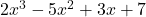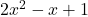One of the critical operations in Algebra is division of polynomials. The four terms involved in it are: Dividend, Divisor, Quotient and Remainder. They are related as follows:

Dividend = Divisor*Quotient + Remainder

We continue the division till the time when degree of remainder becomes less than the dividend.

If remainder is 0, we say that dividend is “completely” divisible by divisor. In other words, Divisor is a factor of Dividend.

Methods:

1. Long division: This is a traditional method which can divide polynomials of any degree

2. Synthetic division: Its younger brother is Synthetic division. This is a tremendously fast method which can give quick results. It works only using constants and not variables. Here is how it works:

Procedure:

To divide a polynomial by a divisor of the formusing synthetic division:

Let the divisor beand dividend beStep 1: Drop down all the constants and make a setup as shown. Notice that the sign of constant on divisor is changed.

Step 2: Drop down the first constant as it as.

Step 3: Multiple this number with the constant over to the left. The result is add to the second term.

Step 4: Keep repeating this process till the last term

Notice the last number? That is the remainder!!

What about the quotient? The first 3 numbers are the coefficient of quotient with one degree less than the dividend.

So, quotient =I am sure you will agree that this is a relatively fast method.

Limitations:

1. It can be used with only linear divisors.

2. Divisor must be of the formI have covered various questions in this video.